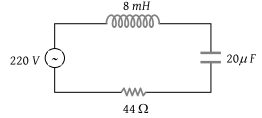# NEET Physics Alternating Current Questions SolvedFor the series LCR circuit shown in the figure, what is the resonance frequency and the amplitude of the current at the resonating frequency(1) 2500 rad – s–1 and $5\sqrt{2}\text{\hspace{0.17em}}A$

(2) 2500 rad – s–1 and 5A

(3) 2500 rad – s–1 and $\frac{5}{\sqrt{2}}A$

(4) 25 rad – s–1 and $5\sqrt{2}\text{\hspace{0.17em}}A$

(2) Resonance frequency

$\omega =\frac{1}{\sqrt{LC}}=\frac{1}{\sqrt{8×{10}^{-3}×20×{10}^{-6}}}=2500\text{\hspace{0.17em}}rad/\mathrm{sec}$

Resonance current = $\frac{V}{R}=\frac{220}{44}=5A$

Difficulty Level:

• 24%
• 50%
• 22%
• 6%
Crack NEET with Online Course - Free Trial (Offer Valid Till September 21, 2019)